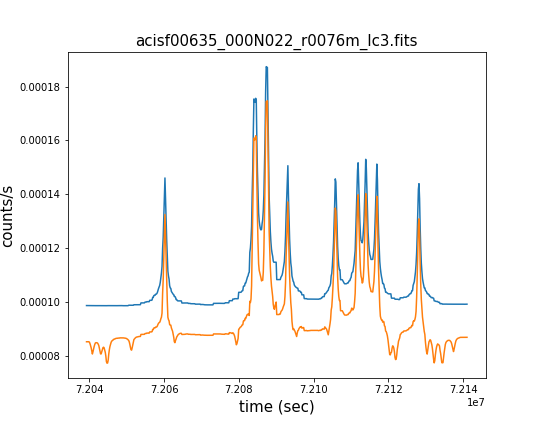## Per-Observation Detections Light Curve Files (lc3.fits)

The light curve files consist of a set of FITS format files, one for each source region of each observation, for each science energy band. Each light curve file has two extensions: the first one (LIGHTCURVE) records the multi-resolution light curve output by the Gregory-Loredo analysis of the arrival times of the source events within the source region. The second extension (BKGLIGHTCURVE) records the background light curve, which has an identical time-binning as the source light curve, but is derived from the events within the background region. Each extensions has the following columns:

• Time — arrival time
• COUNT_RATE — count rate
• COUNT_RATE_ERR — count rate error
• Minus_3Sig — $$3\sigma$$ count rate lower-limit
• Plus_3Sig — $$3\sigma$$ count rate upper-limit

The background light curve is recorded in the FITS HDU (CXC Data Model block) immediately following the source light curve HDUs.

### Source Region Light Curve### Source Region Light Curve

Gregory-Loredo light curves for a source, both before (blue) and after (orange) background subtraction. Background light curve has been scaled prior to subtraction to account for the area difference between the source and background regions.

The Gregory-Loredo method is a Bayesian approach to generating lightcurves on some optimal bin/grid, while smoothing the lightcurve and accounting for the effects of spacecraft dither and detector bad pixels that are not accounted for from standard lightcurves. The smoothing itself is the weighting of the probability of the observed distribution occurring by chance versus the probability of having a constant distribution. How reliable this lightcurve is will be dependent on the Bayesian probabilities calculated. The produced light curve is an estimate of its most probable shape from the source (as opposed to what you just observe with the telescope and instruments).

The per-observation light curve FITS file is named: 〈i〉〈s〉〈obs〉_〈obi〉N〈v〉[_〈c〉]_〈r〉〈b〉_lc3.fits

where 〈i〉 is the instrument designation, 〈s〉 is the data source; 〈obs〉 is the observation identification; 〈obi〉 is the observation interval identification; 〈v〉 is the data product version number; 〈c〉 is the cycle; 〈r〉 is the region ID, formatted as "rnnnn", where nnnn is the 4-digit region number, formatted with leading zeros; and 〈b〉 is the energy band designation. The optional discriminator identified in square brackets is included only for ACIS alternating exposure (interleaved) mode observations.

In CSC 2.0, light curves are computed for each detection at the individual observation level. However, they are not computed if the MLE analysis determines that the observation level detection likelihood is below a threshold that depends on the local background and count rate for the given band. In that case, likelihoods and light curves are not recorded in the database. Consequently, for each source, light curves are only provided for detections that were detected with enough significance.